Important questions of Triangle (in Geometry)

Chapter 6 Class 10 Triangles
Serial order wise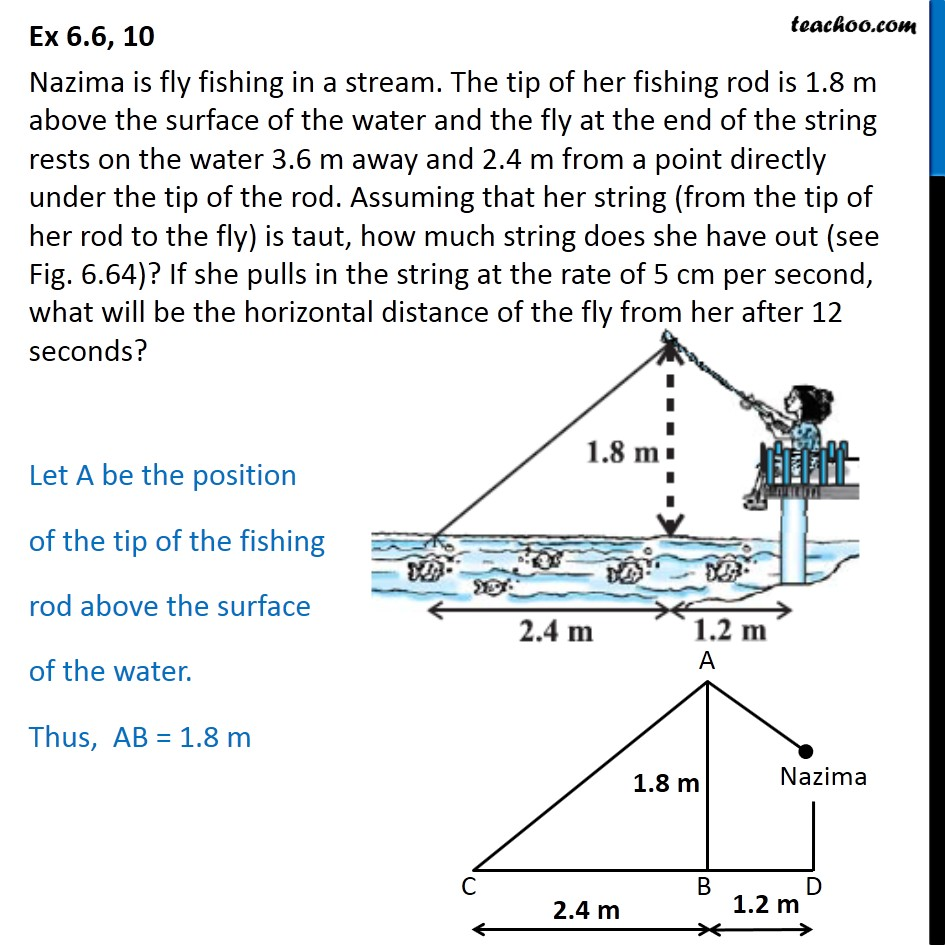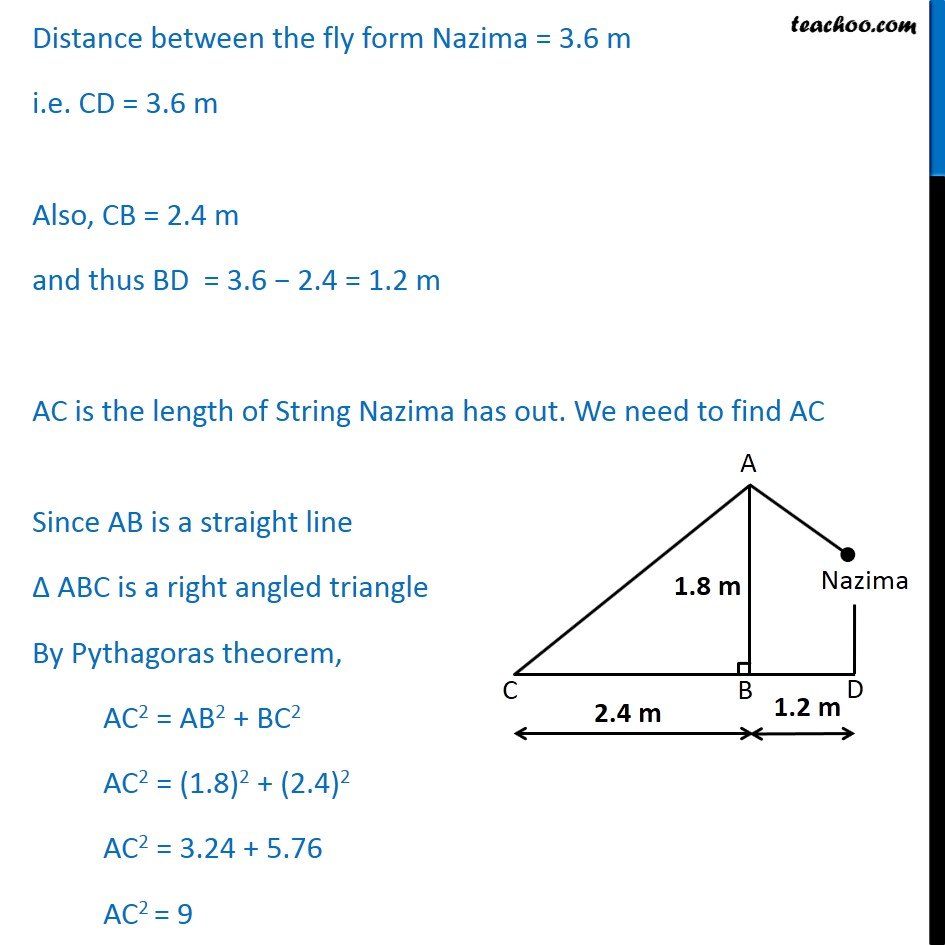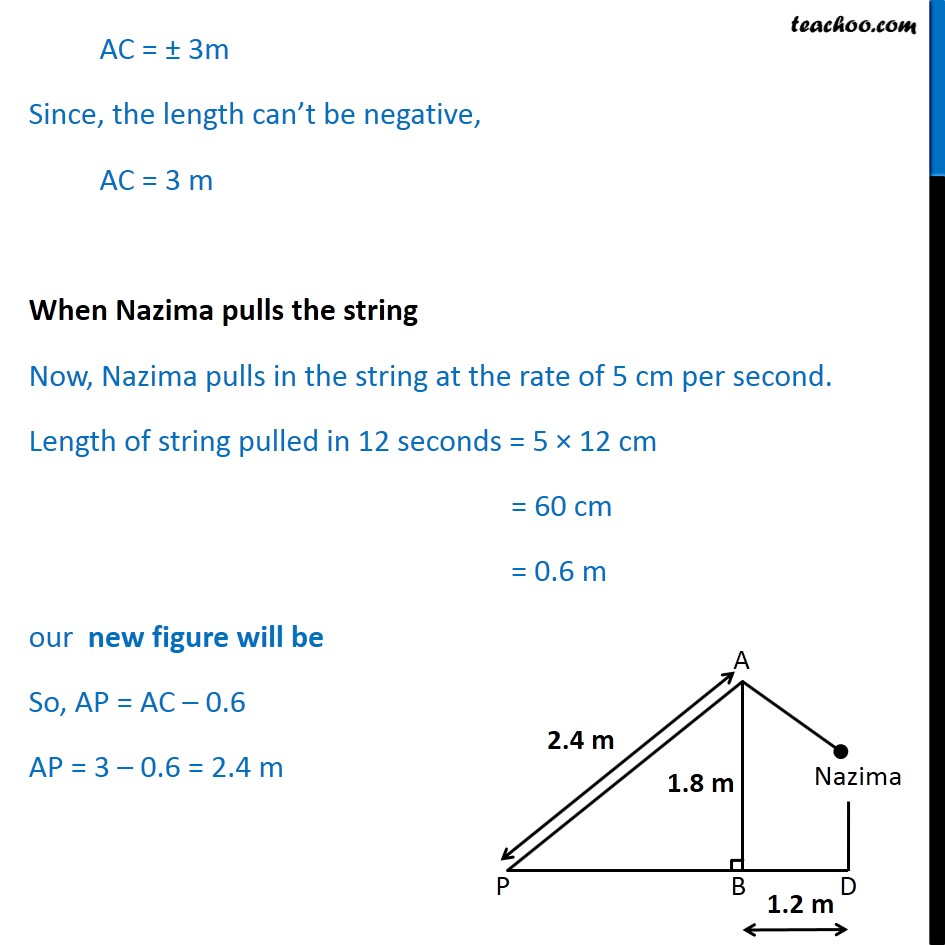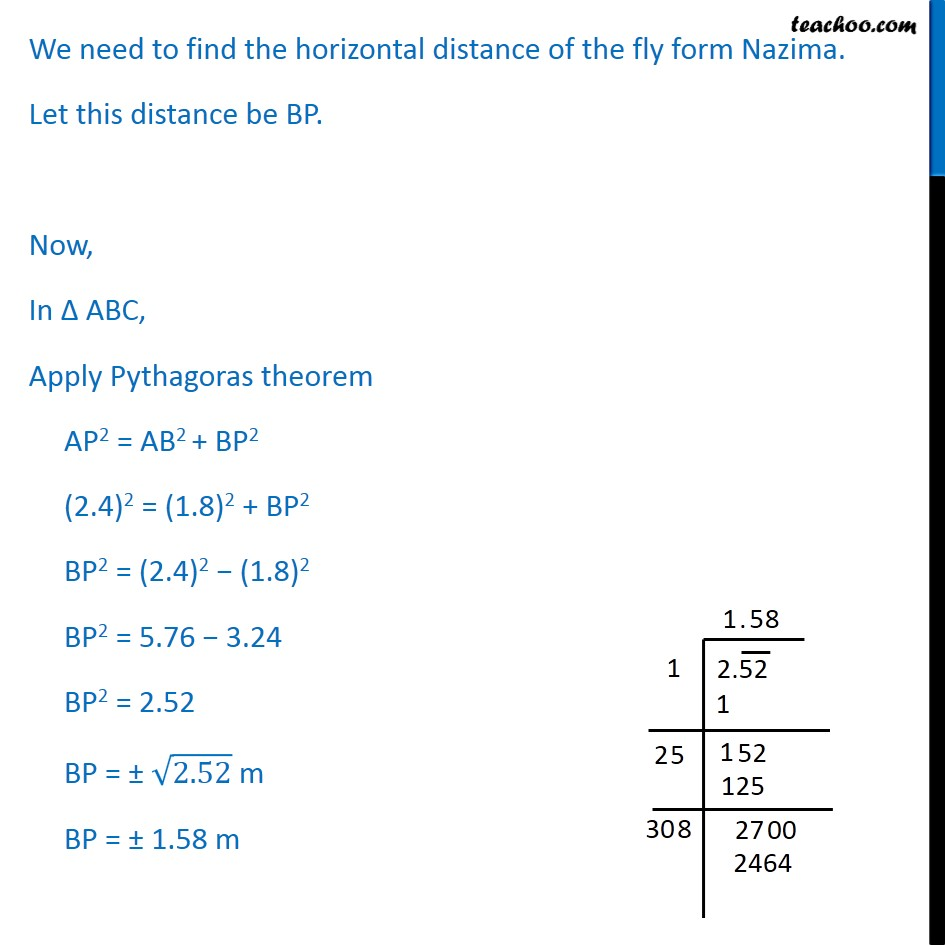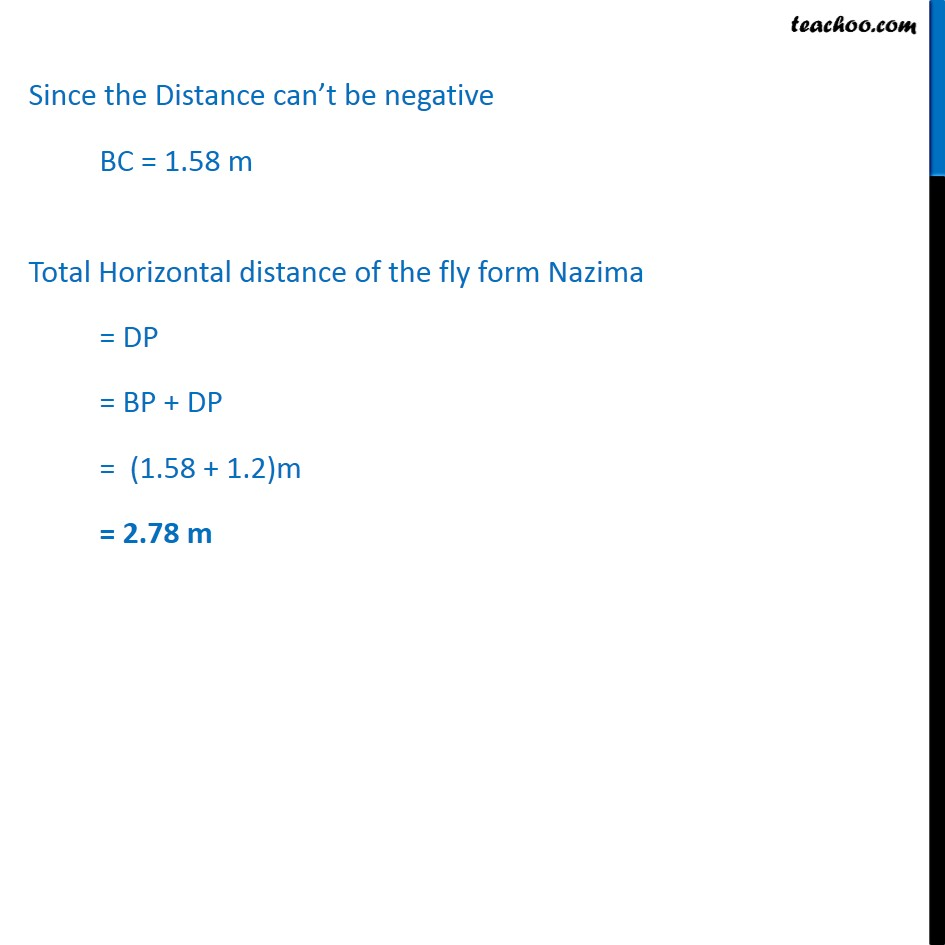Learn in your speed, with individual attention - Teachoo Maths 1-on-1 Class

### Transcript

Question 10 Nazima is fly fishing in a stream. The tip of her fishing rod is 1.8 m above the surface of the water and the fly at the end of the string rests on the water 3.6 m away and 2.4 m from a point directly under the tip of the rod. Assuming that her string (from the tip of her rod to the fly) is taut, how much string does she have out (see Fig. 6.64)? If she pulls in the string at the rate of 5 cm per second, what will be the horizontal distance of the fly from her after 12 seconds? Let A be the position of the tip of the fishing rod above the surface of the water. Thus, AB = 1.8 m Distance between the fly form Nazima = 3.6 m i.e. CD = 3.6 m Also, CB = 2.4 m and thus BD = 3.6 2.4 = 1.2 m AC is the length of String Nazima has out. We need to find AC Since AB is a straight line ABC is a right angled triangle By Pythagoras theorem, AC2 = AB2 + BC2 AC2 = (1.8)2 + (2.4)2 AC2 = 3.24 + 5.76 AC2 = 9 AC = 3m Since, the length can t be negative, AC = 3 m When Nazima pulls the string Now, Nazima pulls in the string at the rate of 5 cm per second. Length of string pulled in 12 seconds = 5 12 cm = 60 cm = 0.6 m our new figure will be So, AP = AC 0.6 AP = 3 0.6 = 2.4 m We need to find the horizontal distance of the fly form Nazima. Let this distance be BP. Now, In ABC, Apply Pythagoras theorem AP2 = AB2 + BP2 (2.4)2 = (1.8)2 + BP2 BP2 = (2.4)2 (1.8)2 BP2 = 5.76 3.24 BP2 = 2.52 BP = 2.52 m BP = 1.58 m Since the Distance can t be negative BC = 1.58 m Total Horizontal distance of the fly form Nazima = DP = BP + DP = (1.58 + 1.2)m = 2.78 m